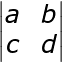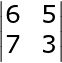# Precalculus Assignment - Trigonometry, Matrices, Limits & Polar Coordinates

Instructor: John Hamilton

John has tutored algebra and SAT Prep and has a B.A. degree with a major in psychology and a minor in mathematics from Christopher Newport University.

The following homeschool assignment covers several mathematical concepts, including trigonometric graphs, matrices, determinants, and the limits of functions. The assignment has been designed for the 12th grade student. Updated: 04/28/2021

## Assignment Explanation and Topic Overview

Did you know the Titanic might have avoided disaster if the crew had a better knowledge of applying polar coordinates to real-world scenarios?

This assignment will juxtapose the Cartesian coordinate system with the polar coordinate system.

By the conclusion of this lesson, your students will have completed four steps, solved three problems, and turned in two relevant presentations.

Note - The answers with detailed explanations are located at the bottom of the page.

## Key Terms

• Determinant: a number computed from a square matrix that can help find the inverse
• Limit: the value a given function approaches in order to determine continuity
• Matrices: arrays of numbers arranged in rectangular or square form

### Materials

• Copy of the unit circle
• Graph paper
• Internet access
• Paper
• Pencil
• Polar coordinate graph paper (optional)
• Protractor
• Ruler

### Time / Length

• Two days to complete the work in this precalculus assignment
• Two weeks to deliver two quality presentations to a teacher and/or class

## Assignment Instructions for Students

### Step One

Let's get started! What are some actual applications of trigonometric graphs in the real world? Well, they can be used for studying sound acoustics, population growth, ocean waves, and automobile engines, just to name a few.

The six types of trigonometric graphs we will discuss are the:

• Sine and Cosine

These two graphs look sort of like ocean waves and are similar to one another, but only the sine graph goes through the point (0, 0).

Example # 1:

Let's graph a sine wave in radians.

If x equals -2π or -6.28, then y equals 0

If x equals -3π/2 or -4.71, then y equals 1

If x equals -π or -3.14, then y equals 0

If x equals -π/2 or -1.57, then y equals -1

If x equals 0, then y equals 0

If x equals π/2 or 1.57, then y equals 1

If x equals π or 3.14, then y equals 0

If x equals 3π/2 or 4.71, then y equals -1

If x equals 2π or 6.28, then y equals 0

Now connect your points by drawing a smooth and curving continuous line. Well done!

• Secant and Cosecant

These two graphs look sort of like a series of parabolas, with the ones above the x-axis opening upwards, and the ones below the x-axis opening downwards.

• Tangent and Cotangent

These two graphs look sort of like a series of curving lines, with the tangent lines appearing to move in a positive direction, one of which passes through the point (0, 0), while the cotangent lines appear to move in a negative direction.

Now go to the Desmos or another online graphing calculator and type in:

• y = sin x
• y = cos x
• y = sec x
• y = csc x
• y = tan x
• y = cot x

Now, do you clearly see the difference between the six functions? Well done!

### Step Two

#### Part 1

By the way, remember your Cartesian coordinate system in which we have a point (x, y)?

Well, what if I told you there existed a similar polar coordinate system in which we have a point (r, θ)?

''Oh wow! Is this like one of those cool science fiction movies in which there exist two alternate, but similar worlds?''

Great analogy. Yes, in fact, you can even convert between the two methodologies.

Example # 2:

Convert point (5, 12) to polar coordinates.

First, find your r with this formula:

r = √(x2 + y2)

r = √ (52 + 122)

r = √ (25 + 144)

r = √ 169

r = 13

Next, find your theta with this formula:

θ = tan-1 (y/x)

θ = tan-1 (12/5)

θ = 67 degrees

Therefore, you have converted your point (5, 12) to the point (13, 67 degrees).

Now you go ahead and try one.

Problem # 1:

Convert point (6, 8) to polar coordinates.

#### Part II

Now let's demonstrate how to graph in polar coordinates. It's not too complicated. First, you just need to remember you will be graphing on a circular graph instead of on a rectangular graph. Second, you need to remember positive is counterclockwise (to the left) and negative is clockwise (to the right) on your graph.

Okay, do you have your graph paper, protractor, and ruler handy? Of course, this will be even easier if you have polar coordinate graph paper. If not, then simply draw a series of concentric circles on your regular graph paper.

Graph the following polar coordinate points:

• (5, 45 degrees)

Count five spaces to your right along the x-axis, and then use your protractor along with your ruler to create a line at a 45 degree angle from the x-axis. In Quadrant I, go ahead and plot your point A.

• (3, -75 degrees)

Count three spaces to your right along the x-axis, and then use your protractor along with your ruler to create a line at a 75 degree angle from the x-axis. However, since you have a negative sign, you will be drawing this line in Quadrant IV instead. Plot your point B.

• (-4, 30 degrees)

This is a bit trickier. Since you have a negative sign, count four spaces to your left along the x-axis, and then use your protractor along with your ruler to create a line at a 30 degree angle from the x-axis. Please note this will be in Quadrant III, as you move counterclockwise for a positive number.

You get the idea. It's not much different from plotting rectangular coordinates, but you can get a bit confused on which way to move along your circles.

### Step Three

Let's learn to determine the limit of a function.

For example, suppose we are asked to find the limit of the function:

Limit ((x - 3) ((x + 2)) / (x - 3)

As x → 3

Well, immediately we know our function is not continuous at x = 3, because when we plug 3 into the equation, we get 0/0 and we can't divide by zero (even when there is also a zero in the numerator).

Looking more closely at this, we see we can actually cancel part of our numerator with our denominator, which results in:

Limit x + 2

As x → 3

Now we simply plug in 3 to get our answer of 5.

Problem # 2:

Evaluate:

Limit (√x - 3) / (x - 9)

As x → 9

### Step Four

Lastly, the term matrix refers to an array of numbers that is used to help solve systems of equations sometimes involving real-world situations.

First, let's solve to obtain the determinant of a matrix, which is a scalar value that provides us with relevant information.

Let's say you are given the 2 x 2 square matrix:a * d - b * c

Problem # 3:

Given the square matrix:Find the determinant.

## Deliverables

You can choose either Option A or Option B to demonstrate your command of this material.

To unlock this lesson you must be a Study.com Member.

### Register to view this lesson

Are you a student or a teacher?